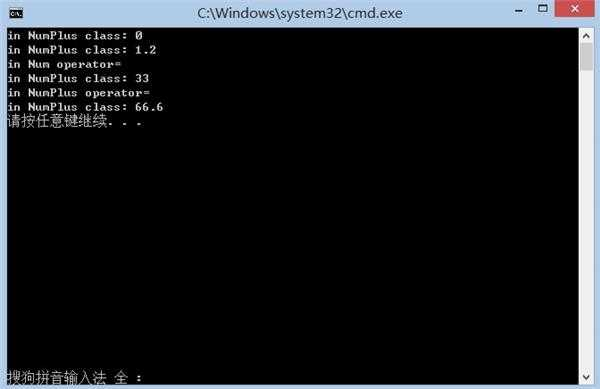﻿ 运算符重载函数设置成虚函数能否有效 | Code Bye

# 运算符重载函数设置成虚函数能否有效

5年前 (2017-04-10) 2644次浏览
本人在VS2015中编写了一个简单的C++程序来测试虚函数的运行情况。结果发现将运算符重载函数设置成虚函数是没有效果的（即程序根据引用或指针的类型来选择调用哪个类的方法，而不是根据引用或指针所指向的对象的实际类型来选择调用哪个类的方法）。

```#include <iostream>
using namespace std;
class Num
{
protected:
int num;
public:
Num(int n = 0);
virtual void show(void) const { cout << "in Num class: " << num << endl; }
virtual Num & operator=(const Num &);
};
class NumPlus : public Num
{
private:
double decmals;
public:
NumPlus(int n = 0, double d = 0.0);
virtual void show(void) const { cout << "in NumPlus class: " << num + decmals << endl; }
virtual NumPlus & operator=(const NumPlus &);
};
Num::Num(int n)
{
num = n;
}
/*本人定义的赋值运算符函数，仅做测试用*/
Num & Num::operator=(const Num & n)
{
cout << "in Num operator=\n";
num = 33;
return (*this);
}
NumPlus::NumPlus(int n, double d) : Num(n)
{
decmals = d;
}
/*本人定义的赋值运算符函数，仅做测试用*/
NumPlus & NumPlus::operator=(const NumPlus & np)
{
cout << "in NumPlus operator=\n";
num = 66;
decmals = 0.6;
return (*this);
}
int main(void)
{
NumPlus a;
a.show();
NumPlus b(1, 0.2);
b.show();
Num & ra = a;
Num & rb = b;
ra.operator=(b); //实际上使用了Num::operator=();
ra.show(); //使用了NumPlus::show();
a.operator=(b);
ra.show();
return 0;
}```

Num::operator=()和NumPlus::operator=()是本人定义的，只是将数据成员赋值成固定的值，仅仅用来测试。20

30

5

CodeBye 版权所有丨如未注明 , 均为原创丨本网站采用BY-NC-SA协议进行授权 , 转载请注明运算符重载函数设置成虚函数能否有效

[1034331897@qq.com]